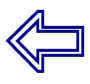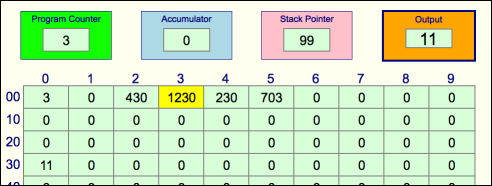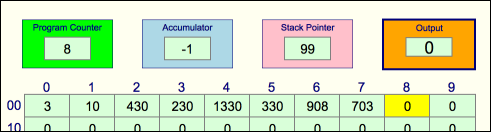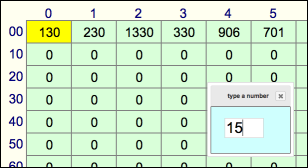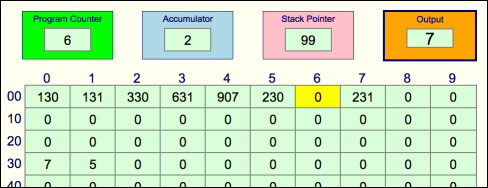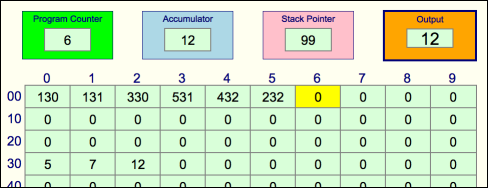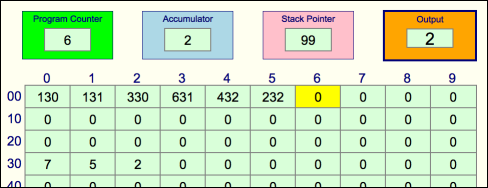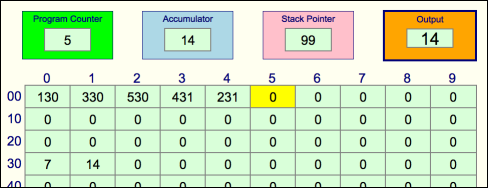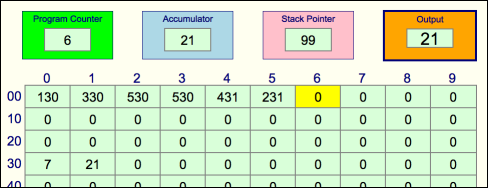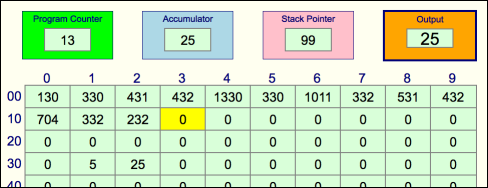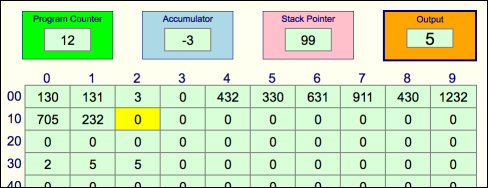Task 5. Create a program to request two numbers from the user and output the larger of the two numbers.

example:  7 5 → 7

Task 6. Create a program to request two numbers from the user and output the sum of the two numbers.

example:  5 7 → 12

Task 7. Create a program to request two numbers from the user and output the difference between the two numbers.

example:  7 5 → 2

Task 8. Create a program to request one number from the user and output its double.

example:  7 → 14

Task 9. Create a program to request one number from the user and output its triple.

example:  7 → 21

Task 10. Create a program to request two numbers from the user and output the product of the two numbers.

example:  5 7 → 35

Task 11. Create a program to request one number from the user and output its square.

example:  5 → 25

Task 12. Create a program to request two numbers from the user and output the result of dividing the first number by the second.

example:  27 5 → 5

You might be interested to see how the simplest of programs can make a computer lose its way completely!. Try:

701 802 701